# GMAT Math : DSQ: Graphing an ordered pair

## Example Questions

### Example Question #30 : Graphing

What quadrant contains the point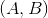, where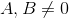?

Statement 1: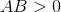Statement 2: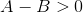BOTH statements TOGETHER are insufficient to answer the question.

EITHER statement ALONE is sufficient to answer the question.

Statement 1 ALONE is sufficient to answer the question, but Statement 2 ALONE is NOT sufficient to answer the question.

BOTH statements TOGETHER are sufficient to answer the question, but NEITHER statement ALONE is sufficient to answer the question.

Statement 2 ALONE is sufficient to answer the question, but Statement 1 ALONE is NOT sufficient to answer the question.

BOTH statements TOGETHER are insufficient to answer the question.

Explanation:

Statement 1 alone tells you thatandare of the same sign, so the point is in Quadrant I (both positive) or Quadrant III (both negative).

Statement 2 tells you that any of the following hold:is positive andis negative - example: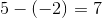is negative andis negative - example: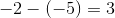is positive andis positive - example: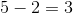This places the point in any quadrant except Quadrant II (whereis negative andis positive).

The two statements together only eliminate two quadrants and leave both Quadrant I and Quadrant III as possibilities.

### Example Question #31 : Graphing

Graph the point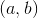.

I)is in quadrant IV.

II)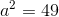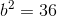.

Statement II is sufficient to answer the question, but statement I is not sufficient to answer the question.

Either statement is sufficient to answer the question.

Both statements are needed to answer the question.

Statement I is sufficient to answer the question, but statement II is not sufficient to answer the question.

Both statements are needed to answer the question.

Explanation:

Graph the point (a,b)

I) (a,b) is in quadrant 4

II)To graph (a,b) we need to know a and b

I) Tells us which quadrant the point is in. In quadrant 4, the x value is positive and the y value must be negative.

II) Lets us find the following: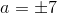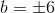So the only possible location ofis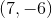.

Therefore, both statements are needed to answer the question.

Tired of practice problems?

Try live online GMAT prep today.